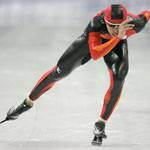Resistance of the air in skating (roller or ice)

For a skater, the resistance of the air increases with the speed.
This resistance does not depend on the skater weight but depends on several parameters not always well perceived.

Skater's speed

The resistance of the air Ra is proportional to the square of the speed relative to the air Va.
Therefore, going twice as fast requires eight more power (cube of the speed).
Furthermore, the speed relative to the air means that with front wind, it is necessary to do an effort identical to the one consisting to go without wind in a speed equal to the skater's speed plus the wind speed.

Weather conditions

The resistance of the air is proportional to the air density ρ which depends on the weather conditions.
It is lower when the air is warm or when the atmospheric pressure is low (bad weather, high altitude).

Aerodynamic profile

The resistance of the air is proportional to the frontal surface of the skater S and the coefficient of aerodynamic shape Cx.
The frontal surface depends on the morphology (size, shoulder width, etc.), but essentially the position of the skater.
The coefficient of aerodynamic shape is proper to the air flow (clothes, etc.).
The product of these two elements defines the penetration coefficient SCx.

 We can consider: SCx = 0.60 for a "vertical" skater SCx = 0.45 for a "60° inclined" skater SCx = 0.35 for a "30° inclined" skater SCx = 0.30 for a "horizontal" skaterCalculation formula

In summary, the mathematic formula allowing calculation is:
Ra = ½ ρ SCx Va²

Calculation of the skating power

J.L